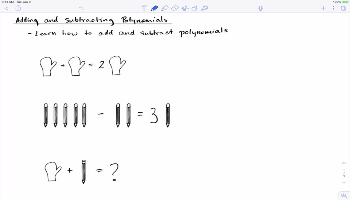### Start Watching Content Today

Sign up for a free LimeSpring account and start watching LimeSpring content today.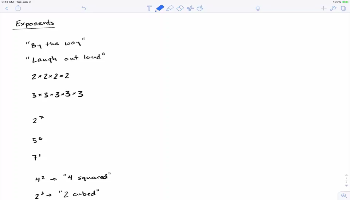##### Exponents

This lesson reviews exponents, including exponent basics, multiplying and dividing numbers and variables raised to exponents, raising a power to a power, the negative power, and the zero power

• Quiz 1
• Quiz 1
• Quiz 1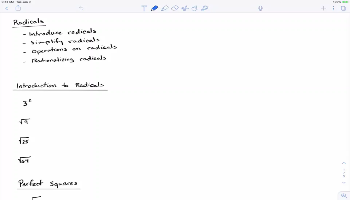• Quiz 1
• Quiz 1
• Quiz 1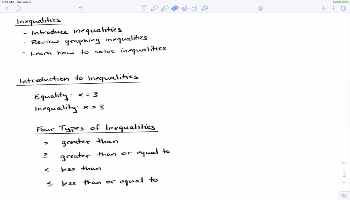##### Inequalities

This lesson introduces inequalities and then discusses graphing and solving inequalities

• Quiz 1
• Quiz 1
• Quiz 1##### Inequalities: Part 2

This lesson dives deeper into inequalities including discussing compound inequalities, absolute value inequalities, and interval notation

• Quiz 1
• Quiz 1
• Quiz 1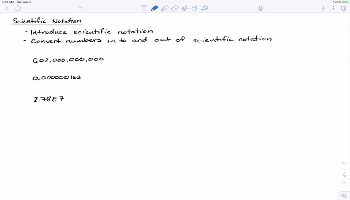##### Scientific Notation

This lesson introduces scientific notation and then discusses how to convert numbers into and out of scientific notation

• Quiz 1
• Quiz 1
• Quiz 1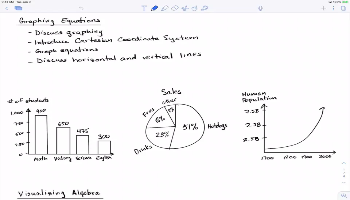##### Graphing Equations

This lesson introduces graphing and the Cartesian Coordinate System, and then delves into graphing linear equations, including vertical and horizontal lines

• Quiz 1
• Quiz 1
• Quiz 1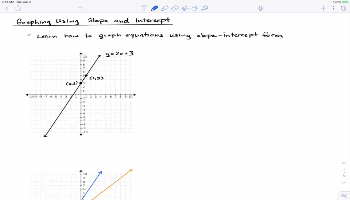##### Graphing Equations Using Slope & Intercept

This lesson introduces the slope-intercept form of a line and then describes how to graph equations using slope and intercept

• Quiz 1
• Quiz 1
• Quiz 1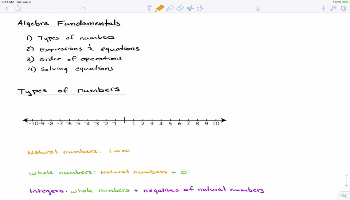##### Algebra Fundamentals

This lesson provides a review of fundamental concepts of algebra including types of numbers, simplifying expressions using the Order of Operations, and solving equations

• Quiz 1
• Quiz 1
• Quiz 1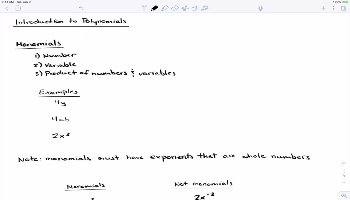##### Introduction to Polynomials

This lesson introduces polynomials and discusses monomials, binomials and trinomials. After that, the lesson talks about the degree of a polynomial and describes general convention for ordering the terms in a polynomial.

• Quiz 1
• Quiz 1
• Quiz 1